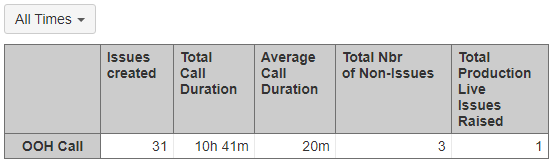# Calculated member not returning data when changing time dimension

Hi,

I’m new to EazyBI and would really appreciate some help.
I have setup the below calculated members to retrieve data from some custom fields. One counts the amount of time and the other counts the average duration.

Amount of Time

``````sum([Measures].[Call Duration due])*60
``````

Average Duration

``````sum([Measures].[Call Duration due]) / [Measures].[Issues created] *60
``````

Example of TableThe issue I’m getting is when I change the time from all time I lose the data in these members.
What do I need to add so that when I change the time dimension it looks and counts that specific time period i.e. Jan 2023.